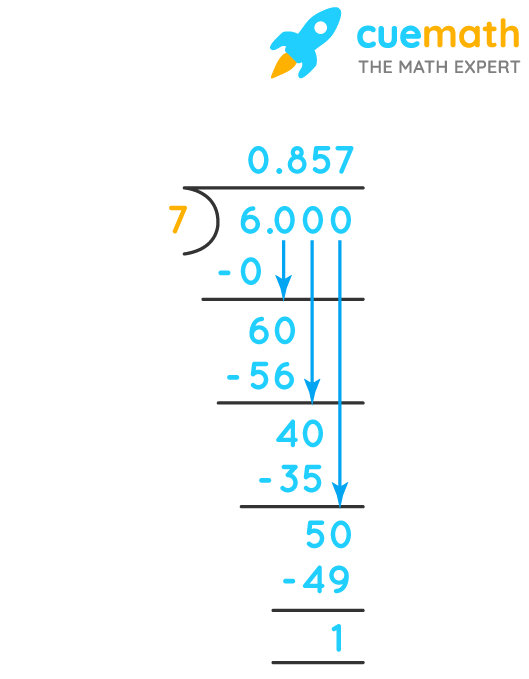# 6/7 As A Decimal

## Question: What is 6/7 as a decimal?

The fractions and decimals are just two ways to represent the same numbers.

## Answer: 6/7 as a decimal is equal to 0.857.

Let's convert the given fraction to a decimal.

## Explanation:

To convert any fraction to decimal form, we just need to divide its numerator by denominator.

Here the fraction is 6/7 which means we need to perform 6 ÷ 7.Therefore, 6/7 = 0.857